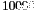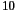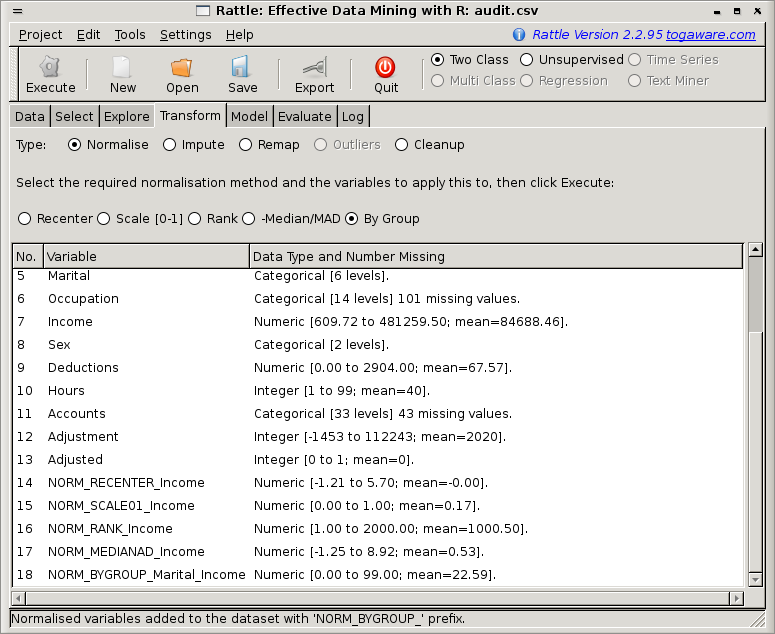DATA MINING
Desktop Survival Guide
by Graham Williams# Rescale Data

Different model builders require different characteristics of the data from which the models will be built. For example, when building a clustering using any kind of distance measure, we may need to normalise the data. Otherwise, a variable like Income will overwhelm a variable like Age, when calculating distances. A distance of 10 ``years'' may be more significant than a distance of \$10,000, yet,swampswhen they are added together, as would be the case by calculating distances.

In these situations we will want to Normalise our data. The types of normalisations (available through the Normalise option of the Transform tab) we may want to perform include re-centering and rescaling our data to be around zero (Recenter), rescaling our data to be in the range from 0 to 1 (Scale [0,1]), covert the numbers into a rank ordering (Rank), and finally, to do a robust rescaling around zero using the median (-Median/MAD). Figuredisplays the interface.We can see in Figure 23.2 the approach we take to normalising (and to transforming) our data. The original data is not modified. Instead, a new variable is created with a prefix added to the variable's name that indicates the kind of transformation. As we can see in the figure, the prefixes are `NORM_RECENTER_`, `NORM_SCALE01_`, `NORM_RANK_`, and `NORM_MEDIANAD_`.

We can see the effect of the four normalisations in comparing the histogram of the variable, Age, in (REPLACE WITH INCOME?) Figure, with the four plots in Figure 23.4 for the corresponding four normalisations.

Subsections